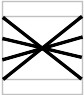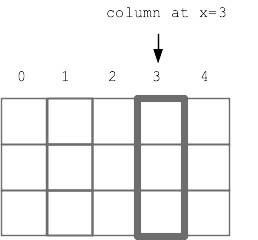# CS106A Midterm Solution

This was a challenging exam, and overall we are happy with how well people did. In any case, we will curve the grades. The median was 75.For people who did poorly, say 45 points or lower, if they do better on the final than on the midterm, we will count the final more and the midterm less in the final grade computation. The final exam will be cumulative, combining all the topics of our 10 weeks.

### Instructions

• There are 7 problems to complete
• In Bluebook, "Problem 1" is these instructions and reference code
• Problems 2-8 are the actual problems
• This is a closed note exam
• The exam is 50 minutes and 100 points, graded on a curve
• We will not grade off for syntax errors, so long as we see the correct idea
• We will not grade off if you omit the final "return" at the end of a function, so long as the code computes the correct result
• Good luck!
```# CS106A Exam Reference Reminder
# [square brackets] denote functions listed here that we have not used yet
# Exam questions will not depend on functions we have not used yet
General functions:
len() int() str() range() list() reversed() [sorted()]
String functions:
isalpha() isdigit() isspace() isupper() islower()
find() upper() lower() [strip()]
List functions:
append() extend() pop() index() [insert()]

SimpleImage:
image = SimpleImage(filename)
# create blank image
image = SimpleImage.blank(width, height)

# foreach loop
for pixel in image:

# range/y/x loop
for y in range(image.height):
for x in range(image.width):
pixel = image.get_pixel(x, y)

Canvas Draw
canvas.draw_line(x1, y1, x2, y2)
# x,y is upper left, optional color='red' parameter
canvas.draw_rect(x, y, width, height)
canvas.fill_rect(x, y, width, height)
canvas.draw_oval(x, y, width, height)
canvas.fill_oval(x, y, width, height)

Grid 2D:
grid = Grid.build([['row', '0'], ['row', '1']])
grid.width, grid.height - properties
grid.in_bounds(x, y) - True if in bounds
grid.get(x, y) - returns contents at that x,y, or None if empty
grid.set(x, y, value) - sets new value into grid at x,y

Read lines out of a text fie:
with open(filename) as f:
for line in f:
```

### 2. Short Answer (2 points)

What 3 numbers print when the caller() function runs? This code runs without error.

```def foo(a):
b = 1
a = a + b
return a

def caller():
a = 1
b = 2
c = foo(b)
print(a, b, c)
```
```# Write 3 numbers here

```

### 3. Short Strings (8 points)

Write code for the two functions:

`reverse(s):` our old friend, this function. Given string s, return a string with the chars of s in reverse order, so `'Hello'` returns `'olleH'`.

`alldigits(s):` Given a string s, treat every digit in s as an int value 0..9. Return a list of all these int values parsed from s. So e.g. `'ab3x4x22'` returns `[3, 4, 2, 2]`. This can be done with a simple for-loop.

```def reverse(s):
pass

def alldigits(s):
pass
```

### 4. Double Encrypt (20 points)

This problem is similar to the Crypto homework with the simplification that the encryption is done entirely with lowercase chars.

This "double" encryption uses two source lists, src1 and src2, and two slug lists, slug1 and slug2. All the lists contain chars and are the same length. Here is the double_encrypt() algorithm: use the lowercase version of the input char, which may be uppercase. If the char is in src1, return the char at the corresponding index in slug1.

If the char is in src2, return the char at the corresponding "reverse index" in slug2, e.g. like this for lists length 4:

```index  reverse-index
0       3
1       2
2       1
3       0
```

If the char is not in src1 or src2, return the char unchanged. No char appears in both src1 and src2.

```Double Encrypt Example (len 4)
src1  = ['a', 'b', 'c', 'd']
slug1 = ['s', 't', 'u', 'v']
src2  = ['e', 'f', 'g', 'h']
slug2 = ['w', 'x', 'y', 'z']
encrypt 'A' -> 's'
encrypt 'e' -> 'z'
encrypt 'f' -> 'y'
encrypt '\$' -> '\$'
```
```def double_encrypt(src1, slug1, src2, slug2, char):
pass

```

### 5. Drawing (10 points)

Reminder: the canvas draw_line function:

```canvas.draw_line(x1, y1, x2, y2)
- draws a line from x1,y1 to x2,y2
```

As on the Quilt homework, the function will take in the x,y width,height of a figure positioned on the canvas, and draw lines within that figure.

Leave a 10-pixel high margin across the top and bottom of the figure without any drawing. Given int n which is 2 or more, draw n lines, as follows: The first line should begin at the left edge, 10 pixels from the top, and extend to the right edge, 10 pixels from the bottom. The last line should start at the left edge, 10 pixels from the bottom, and extend to the right edge 10 pixels from the top, with the other lines distributed proportionately.

Here is a picture showing the drawing for n=4 lines. The 4 lines to draw are shown as heavy black lines, with the outer edge of the figure and the margin areas outlined in gray.```def draw(canvas, x, y, width, height, n):
pass

```

### 6. Grid (20 points)

For this problem you will implement a new operation that works on one vertical column of squares in the grid. Every square in the grid is either a rock 'r', sand 's', or empty None.

The jumpdown(grid, x, n) algorithm works as follows: The parameter x will be an in-bounds x value, indicating the column to work on. The parameter n will be 1 or more, the number of squares down to move each sand.Look at every "candidate" square in the column, working from the bottom of the column to the top. If the candidate square contains sand, the sand will be moved. Its "destination" square is n squares straight down, (n=1 meaning the square immediately below). If the destination is empty, move the candidate sand there. If the destination is not empty or is out of bounds, then erase the candidate sand from the grid. Obstacles between the candidate sand and its destination do not matter - the sand jumps to its destination.

```>>> grid = Grid.build([['s', 's'], ['r', None], [None, 'r']])
>>> jumpdown(grid, 0, 2)
[[None, 's'], ['r', None], ['s', 'r']]
>>> grid = Grid.build([['s', 's'], ['r', None], [None, 'r']])
>>> jumpdown(grid, 1, 2)
[['s', None], ['r', None], [None, 'r']]
>>> grid = Grid.build([['s', None], ['s', None], [None, None]])
>>> jumpdown(grid, 0, 1)
[[None, None], ['s', None], ['s', None]]
```
```def jumpdown(grid, x, n):
pass

```

### 7. Nesting (15 points)

Given a non-negative int n. Create and return a list containing n nested lists. The first nested list is `[1, 2]`. The last nested list is `[1, 2, 3, ... 2 * n]`

```n = 0 ->
[]

n = 1 ->
[[1, 2]]

n = 2 ->
[[1, 2], [1, 2, 3, 4]]

n = 3 ->
[[1, 2], [1, 2, 3, 4], [1, 2, 3, 4, 5, 6]]
```
```def nesting(n):
pass

```

### 8. Parse (25 points)

We'll say a "digalpha" word is a sequence of chars starting with 1 digit, followed by 1 or more alphabetic chars. So for example the digalpha words in
`'1abc xx 2y 6 34 56hi'` are `['1abc', '2y', '6hi']`

Write nested-loop code to build and return a list of all the digalpha words in string s. (Some boilerplate code provided to start)

```'1abc xx 2y 6 34 56hi' -> ['1abc', '2y', '6hi']
'abc1x abc x 2 aa3bb' -> ['1x', '3bb']
'123 abc 55' -> []
```
```def digalpha(s):
search = 0
result = []
while True:
pass

```

### Soutions

```1, 2, 3

def reverse(s):
result = ""
for i in reversed(range(len(s)):
result += s[i]
return result

def alldigits(s):
lst = []
for ch in s:
if ch.isdigit():
lst.append(int(ch))
return lst

def encrypt_double(src1, slug1, src2, slug2, char):
"""
>>> # Make len-4 lists for testing
>>> src1  = ['a', 'b', 'c', 'd']
>>> slug1 = ['s', 't', 'u', 'v']
>>> src2  = ['e', 'f', 'g', 'h']
>>> slug2 = ['w', 'x', 'y', 'z']
>>> encrypt_double(src1, slug1, src2, slug2, 'A')
's'
>>> encrypt_double(src1, slug1, src2, slug2, 'e')
'z'
>>> encrypt_double(src1, slug1, src2, slug2, 'f')
'y'
>>> encrypt_double(src1, slug1, src2, slug2, '\$')
'\$'
"""
lower = char.lower()
if lower in src1:
idx = src1.index(lower)
return slug1[idx]
if lower in src2:
idx = src2.index(lower)
rev = len(src2) - idx - 1
return slug2[rev]
return char

def draw(canvas, x, y, width, height, n):
for i in range(n):
y_add = i/(n - 1) * (height - 20 - 1)
canvas.drawline(x, y + 10 + y_add,
x + width - 1, y + height - 1 - 10 - y_add)

def jumpdown(grid, x, n):
"""
>>> grid = Grid.build([['s', 's'], ['r', None], [None, 'r']])
>>> jumpdown(grid, 0, 2)
[[None, 's'], ['r', None], ['s', 'r']]
>>> grid = Grid.build([['s', 's'], ['r', None], [None, 'r']])
>>> jumpdown(grid, 1, 2)
[['s', None], ['r', None], [None, 'r']]
>>> grid = Grid.build([['s', None], ['s', None], [None, None]])
>>> jumpdown(grid, 0, 1)
[[None, None], ['s', None], ['s', None]]
"""
for y in reversed(range(grid.height)):
if grid.get(x, y) == 's':
grid.set(x, y, None)
dest_y = y + n
if grid.in_bounds(x, dest_y):
if grid.get(x, dest_y) == None:
grid.set(x, dest_y, 's')
return grid

def nesting(n):
"""
>>> nesting(3)
[[1, 2], [1, 2, 3, 4], [1, 2, 3, 4, 5, 6]]
"""
outer = []
for num in range(1, n + 1):
inner = []
for i in range(1, 2 * num + 1):
inner.append(i)
outer.append(inner)
return outer

def digalpha(s):
"""
>>> digalpha('1abc xx 2y 34 56hi')
['1abc', '2y', '6hi']
>>> digalpha('abc1x abc x 2 aa3bb')
['1x', '3bb']
>>> digalpha('123 abc 55')
[]
"""
search = 0
result = []
while True:
start = search
while start < len(s) and not s[start].isdigit():
start += 1

if start >= len(s):
break

end = start + 1
while end < len(s) and s[end].isalpha():
end += 1

word = s[start:end]
if len(word) >= 2:
result.append(word)
search = end
return result
```